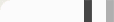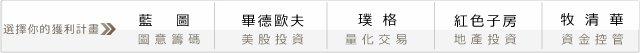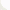## 排在資金控管之前：為什麼你該不斷尋找交易市場?

#### 從一場賭局出發...

2*50% + (-1)*50% = 0.5

[p(1+b)-1]/b = [50%(1+2)-1]/2 = 25%

#### 同時玩兩場賭局: 初窺 Leverage Space Model

Case 1. 賭局A贏，賭局B贏；故資產變為\$100 + \$20*2 + \$20*2 = \$180元
Case 2. 賭局A贏，賭局B輸；故資產變為\$100 + \$20*2 - \$20*1 = \$120元
Case 3. 賭局A輸，賭局B贏；故資產變為\$100 - \$20*1 + \$20*2 = \$120元
Case 4. 賭局A輸，賭局B輸；故資產變為\$100 - \$20*1 - \$20*1 = \$60元

A(f)=[(1+4f)^10]*[(1+f)^10]*[(1+f)^10]*[(1-2f)^10

Case 1. 賭局A贏，賭局B贏，賭局C贏：原資金乘上 (1+2f+2f+2f)=(1+6f)；
Case 2. 賭局A贏，賭局B輸，賭局C贏：原資金乘上 (1+2f-f+2f)=(1+3f)；
Case 3. 賭局A輸，賭局B贏，賭局C贏：原資金乘上 (1-f+2f+2f)=(1+3f)；
Case 4. 賭局A輸，賭局B輸，賭局C贏：原資金乘上 (1-f-f+2f)=1；
Case 5. 賭局A贏，賭局B贏，賭局C輸：原資金乘上 (1+2f+2f-f)=(1+3f)；
Case 6. 賭局A贏，賭局B輸，賭局C輸：原資金乘上 (1+2f-f-f)=1；
Case 7. 賭局A輸，賭局B贏，賭局C輸：原資金乘上 (1-f+2f-f)=1；
Case 8. 賭局A輸，賭局B輸，賭局C輸：原資金乘上 (1-f-f-f)=(1-3f)；

"相關性"這個變量考慮進去。在Kelly公式的延伸上，Vince's Leverage Space Model正是在處理這樣的問題。

#### 排在資金控管之前，英雄慎選"多元"戰場!

In Some Sense，選擇多元市場也是一種資金控管!

#### 0 意見: